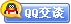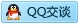##用户名 Email 自动登录 找回密码 密码 会员注册
 VIP会员，3年作业免费下 ！ 奥鹏作业，奥鹏毕业论文检测 新手作业下载教程，充值问题 没有找到答案，请在此处留言！ 2020年04月最新全国统考资料 投诉建议，加盟合作！奥鹏课程积分软件(ver:3.1)

# 吉大15春学期《JAVA程序设计》在线作业一答案辅导资料发表于 2015-4-10 23:16:30 | 显示全部楼层 |阅读模式谋学网(www.mouxue.com)是国内最专业的奥鹏作业答案，奥鹏离线作业答案及奥鹏毕业论文辅导型网站，主要提供奥鹏中医大、大工、东财、北语、北航、川大、南开等奥鹏作业答案辅导，致力打造中国最专业的远程教育辅导社区。 ) ?; C: f4 L3 K8 N5 } 一、单选题（共 15 道试题，共 60 分。）V 1.  int 型 public 成员变量 MAX_LENGTH，该值保持为常数100，则定义这个变量的语句是( )( V% ~- i& `: ?6 r7 s, l  D A. public int MAX_LENGTH=100 B. final int MAX_LENGTH=100: v' O/ T, D' G C. public const int MAX_LENGTH=100 D. public final int MAX_LENGTH=100       满分：4  分 2.  用于定义类成员的访问控制权的一组关键字是( ) A. class, float, double, public2 {1 Q# z6 N3 `1 ]! ]1 | B. float, boolean, int, long- h; c- z2 o9 c" }3 j* y" T C. char, extends, float, double D. public, private, protected& ?8 Y, z/ d8 B) C& F: x       满分：4  分. |: K" H2 Z3 o 3.  下列运算符中，优先级最高的是( )。1 H# O: X: `' a! D0 }( M# B A. && B. ++ C. + D. *       满分：4  分 4.  关于以下程序段的说法正确的是( )。 1. public class Myar{ 2. public static void main(String argv[]){ 3. int[]i=new int; 4. System.out.println(i); 5. } 6. } A. 第2行编译出错 B. 第4行编译出错 C. 编译时产生错误  z5 Z( K; g: p. e7 I% Q D. 编译时没有产生错误，运行时发生异常       满分：4  分' w5 J; O+ t9 X' g* C 5.  在switch(expression)语句中，expression的数据类型不能是( )。2 c) r) }' A* ?8 H& x( U: E A. double/ Z2 T) W4 {& H/ F B. char5 s6 P+ v2 p. I C. byte) J7 d" y& Z: z! u+ \ D. short4 [( @, V1 n, i/ E3 L( f       满分：4  分9 p& I4 p2 O2 |: ^ 6.  若已定义 byte[ ] x= {11,22,33,-66} ; 其中0≤k≤3，则对x数组元素错误的引用是( ) A. x[5-3]4 ?6 n# J, p; z; A B. x[k]* B2 v" H  w1 F! p! W- w9 ~ C. x[k+5] D. x/ e1 ]+ W& i/ u( ^4 }; v       满分：4  分9 h  i" I( j8 Y! T 7.  下列语句序列执行后，ch1 的值是( )。 char ch1='A',ch2='W'; if(ch1 + 2 < ch2 ) ++ch1;! @* O& ^+ T7 n' t0 C A. ‘A' B. B'5 o) Z; Z9 m/ l7 o6 e C. ‘C': z# I' q9 M4 T6 u  k" S D. B& [1 _% V' n4 z       满分：4  分 8.  以下有关类的继承的叙述中，正确的是( )% _. R( ?; X\$ l- @* }\$ [' C A. 子类能直接继承父类所有的非私有属性，也可通过接口继承父类的私有属性 B. 子类只能继承父类的方法，不能继承父类的属性6 V6 u9 u6 t/ Y& Y\$ w C. 子类只能继承父类的非私有属性，不能继承父类的方法 D. 子类不能继承父类的私有属性) b* O\$ K\$ m( e+ O: s       满分：4  分 9.  下列关于for循环和while循环的说法中哪个是正确的( ): k7 y; ?  a- z\$ \1 m A. while循环能实现的操作，for循环也都能实现/ z* m5 W\$ [( w# ? B. while循环判断条件一般是程序结果，for循环判断条件一般是非程序结果 C. 两种循环任何时候都可替换/ g- `) e& Z4 p4 E- A D. 两种循环结构中都必须有循环体，循环体不能为空       满分：4  分 10.  设 i、j 为int型变量名，a 为int型数组名，以下选项中，正确的赋值语句是( ) A. i = i + 2, k& b. W2 k& H2 x: g. ~# t B. a = 7; C. i++ - --j;0 q( H( ]\$ v/ s/ @ D. a(0) = 66;       满分：4  分 11.  以下选项中，合法的赋值语句是( )5 i& E/ B: R1 B& |6 s A. a = = 1;) F4 I1 }. d, j+ l' l/ O1 y# f\$ P* X B. ++ i; C. a=a + 1= 5;( u5 A0 l5 D6 S# [/ t0 t4 n9 M! y: N D. y = int ( i );       满分：4  分: z* }9 v7 \5 L+ g" n* L 12.  要使用Vector必须import入下面哪个包( )。 A. java.Vector4 \; Q4 k\$ T& P  L. e. Q B. java.io( j' j8 e5 w9 { C. java.util D. java.Applet3 l5 D! a2 T* E2 _* S       满分：4  分 13.  设 x = 1 , y = 2 , z = 3，则表达式 y＋＝z－－/＋＋x 的值是( )9 R7 t8 S" j* w! J A. 3 B. 3.5( s. P+ b' k* k1 P8 |; ? C. 4 D. 5       满分：4  分 14.  下列语句序列执行后，k 的值是( )。 int i=10, j=18, k=30; switch( j - i ) { case 8 : k++; case 9 : k+=2; case 10: k+=3; default : k/=j; } A. 31 B. 32 C. 2 D. 33- Q! O\$ {  s+ t3 M8 Z       满分：4  分\$ R( t) R( I# d8 _: l! r 15.  下面的表达式哪个是正确的( ) A. String s="你好";int i=3; s+=i; B. String s="你好";int i=3; if(i==s){ s+=i};% @& p1 X( W# d! h8 ^ C. String s="你好";int i=3; s=i+s;+ S0 w: `  S- O, z D. String s="你好";int i=3; s=i+;       满分：4  分 , h1 B) D! a  ?/ A. w0 F* ~, p! M4 M 二、多选题（共 5 道试题，共 20 分。）V 1.  选出合理的标识符( ) A. _sys1_lll6 F% [\$ o# W\$ d. @( u2 l\$ U B. 2mail C. \$change D. class' u\$ {8 H6 D- p% T       满分：4  分 2.  数组与向量的区别是( )。 A. 数组的长度固定7 Z. A) X! D8 m7 v3 S: G! W B. 数组元素的数据类型相同，可以是普通数据类型 C. 向量长度可变5 M, U: [- v( T5 R; X. e D. 向量的元素类型可不同，只能是对象的引用       满分：4  分 3.  下面哪个说法正确( )。* Z, `0 k+ y7 ]/ f# y4 i4 ^/ ~; |( H A. 如果某公共的接口被声明在一个源文件中，则接口必须与源文件具有相同的名字。; t5 a\$ W6 Q' h0 |2 k0 S B. 如果源文件包含import语句，则该语句必须是除空行和注释行外的第一个语句行。 C. 如果某源文件包含package语句，则该语句必须是第一个非空、非注释行。 D. 接口中的方法必定是抽象方法，但可以不用关键字abstract修饰。- i4 g* d( `3 w9 l       满分：4  分 4.  以下哪些是Java语言的特点( )3 d: s# L' T\$ q& a# y4 d7 P A. 面向对象3 J1 r  E5 R\$ ]+ r3 _ B. 安全可靠 C. 平台无关 D. 多支持线程 E. 支持分布操作. _! l6 x& I; Y" o  e       满分：4  分  w- J6 d# e6 V  M+ s 5.  如下哪些是Java中有效的关键字( ) A. Const B. NULL- h/ B  V; R. m, \ C. false D. this E. native! q* W' x+ }) o: J       满分：4  分 - y) I  [! |0 u; f2 T9 _ + A0 y8 q- \! Q1 E" |1 s+ e9 n 三、判断题（共 5 道试题，共 20 分。）V 1.  子类要调用父类的方法，必须使用super关键字( ); ]% F! `0 U, y2 j7 [/ i A. 错误 B. 正确' t( L( s3 ^9 r       满分：4  分 2.  抽象类里一定要包含抽象方法，但含抽象方法的类未必是抽象类( )。 A. 错误4 w7 [8 ]3 h4 A) a B. 正确* h  @3 A\$ o& S) B) V       满分：4  分 3.  Java的字符类型采用的是Unicode编码，但所占字节由具体软硬件环境决定( ) A. 错误  @\$ Q# P! W8 u4 u. A B. 正确       满分：4  分 4.  如果p是父类Parent的对象，而c是子类Child的对象，则语句c = p是正确的( ) A. 错误" c9 @( T# L5 s; A3 i3 K9 f; [ B. 正确       满分：4  分% w) T- f) R- c% s. g% V  q 5.  Java语言中的数组元素只能是基本数据类型而不能为对象类型( ) A. 错误\$ n% l; w3 y* U* v\$ C& c. U B. 正确8 ~3 `! m% R. H3 T" [       满分：4  分 谋学网(www.mouxue.com)是国内最专业的奥鹏作业答案，奥鹏离线作业答案及奥鹏毕业论文辅导型网站，主要提供奥鹏中医大、大工、东财、北语、北航、川大、南开等奥鹏作业答案辅导，致力打造中国最专业的远程教育辅导社区。

### 本帖子中包含更多资源

x
B Color Image Link Quote Code Smilies |上传客服一客服二客服三客服四微信客服扫一扫